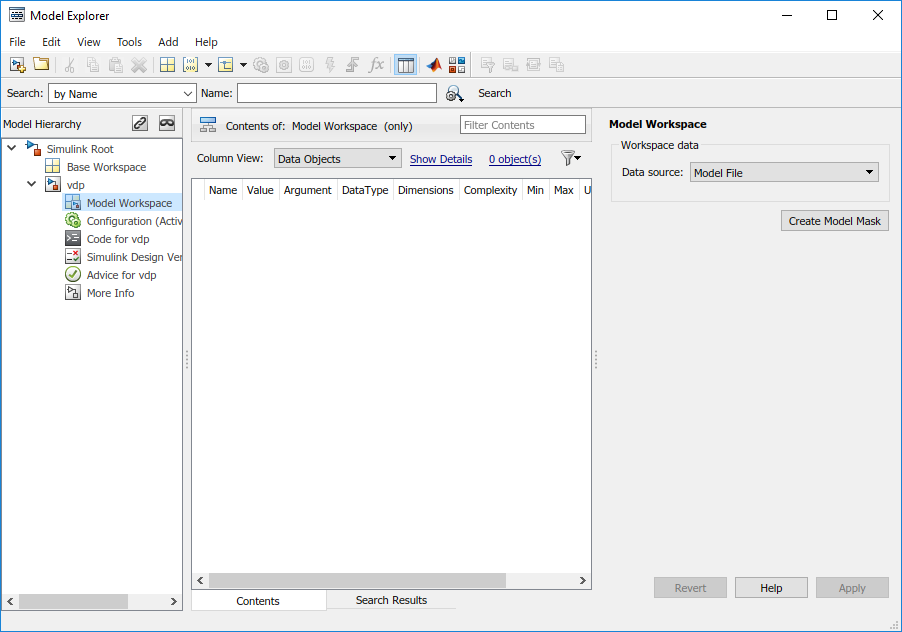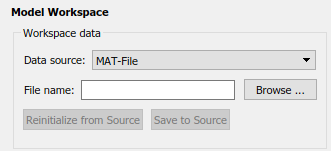Documentation

Specify Source for Data in Model Workspace

When you use a model workspace to contain the variables that a model uses, you can choose to store the variables in one of these sources:

• The model file, which can store static variable definitions.

• A separate MAT-file or MATLAB® file. You can reload the variables from the external file into the model workspace at any time.

• Your own custom MATLAB code that creates variables. You can save the code as part of the model file, and reload the code at any time.

To specify a data source for a model workspace, in the Model Explorer, use the Model Workspace dialog box. To display the dialog box for a model workspace:

1. Open the Model Explorer. On the Modeling tab, click Model Data Editor.

2. In the Model Hierarchy pane, right-click the model workspace.3. Select the Properties menu item, which opens the Model Workspace dialog box.

To use MATLAB commands to change data in a model workspace, see Use MATLAB Commands to Change Workspace Data.

Data source

The Data source field in the Model Workspace dialog box includes the following data source options for a workspace:

• Model File

Specifies that the data source is the model itself.

• MAT-File

Specifies that the data source is a MAT file. Selecting this option causes additional controls to appear (see MAT-File and MATLAB File Source Controls).

• MATLAB File

Specifies that the data source is a MATLAB file. Selecting this option causes additional controls to appear (see MAT-File and MATLAB File Source Controls).

• MATLAB Code

Specifies that the data source is MATLAB code stored in the model file. Selecting this option causes additional controls to appear (see MATLAB Code Source Controls).

MAT-File and MATLAB File Source Controls

Selecting MAT-File or MATLAB File as the Data source for a workspace causes the Model Workspace dialog box to display additional controls.File name

Specifies the file name or path name of the MAT-file or MATLAB file that is the data source for the selected workspace. If you specify a file name, the name must reside on the MATLAB path.

Reinitialize From Source

Clears the workspace and reloads the data from the MAT-file or MATLAB file specified by the File name field.

Save To Source

Saves the workspace in the MAT-file or MATLAB file specified by the File name field.

MATLAB Code Source Controls

Selecting MATLAB Code as the Data source for a workspace causes the Model Workspace dialog box to display additional controls.MATLAB Code

Specifies MATLAB code that initializes the selected workspace. To change the initialization code, edit this field, then select the button on the dialog box to clear the workspace and execute the modified code.

Reinitialize from Source

Clears the workspace and executes the contents of the MATLAB Code field.